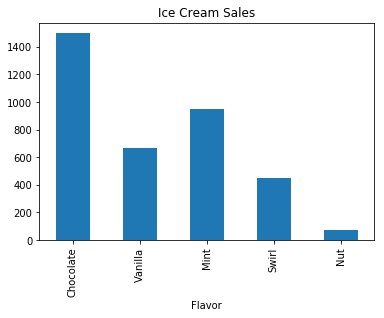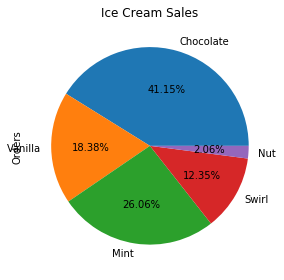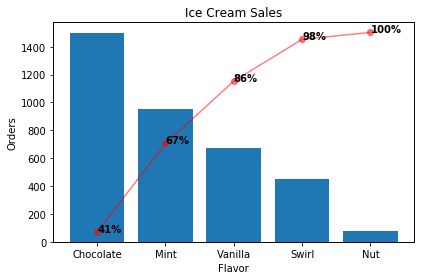# Pareto Plot With Matplotlib

A Pareto plot is essentially a sorted bar chart in descending order that shows the cumulative total via a line. It combines the information of a bar chart and a pie chart into one. In this post I demonstrate how to create a Pareto plot in Python with matplotlib.

In :
import pandas as pd
import seaborn as sns

import matplotlib.pyplot as plt
%matplotlib inline


Create some sample data that is sales from an ice cream shop.

In :
df = pd.DataFrame({
'Flavor': ['Chocolate', 'Vanilla', 'Mint', 'Swirl', 'Nut'],
'Orders': [1500,  670,  950,  450,   75]
})


Plot a bar and pie chart of the data to illustrate the benefit of using a Pareto plot.

In :
df.plot(kind='bar', x='Flavor', y='Orders', legend=None, title='Ice Cream Sales')
plt.show()In :
df.plot(kind='pie', labels=df['Flavor'], y='Orders', legend=None, autopct='%.2f%%', title='Ice Cream Sales')
plt.tight_layout()
plt.show()In :
def pareto_plot(df, x=None, y=None, title=None, show_pct_y=False, pct_format='{0:.0%}'):
xlabel = x
ylabel = y
tmp = df.sort_values(y, ascending=False)
x = tmp[x].values
y = tmp[y].values
weights = y / y.sum()
cumsum = weights.cumsum()

fig, ax1 = plt.subplots()
ax1.bar(x, y)
ax1.set_xlabel(xlabel)
ax1.set_ylabel(ylabel)

ax2 = ax1.twinx()
ax2.plot(x, cumsum, '-ro', alpha=0.5)
ax2.set_ylabel('', color='r')
ax2.tick_params('y', colors='r')

vals = ax2.get_yticks()
ax2.set_yticklabels(['{:,.2%}'.format(x) for x in vals])

# hide y-labels on right side
if not show_pct_y:
ax2.set_yticks([])

formatted_weights = [pct_format.format(x) for x in cumsum]
for i, txt in enumerate(formatted_weights):
ax2.annotate(txt, (x[i], cumsum[i]), fontweight='heavy')

if title:
plt.title(title)

plt.tight_layout()
plt.show()

In :
pareto_plot(df, x='Flavor', y='Orders', title='Ice Cream Sales')From our toy example, we can see that chocolate, mint and vanilla make up 86% of our sales. From an advertising perspective, we could have some sort of discount associated with those flavors to further increase them. Hopefully, you can see the benefit of using this plot instead of a separate bar and pie chart.

Contents © 2020 Tyler Marrs